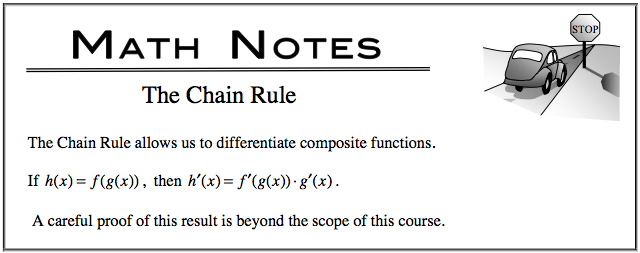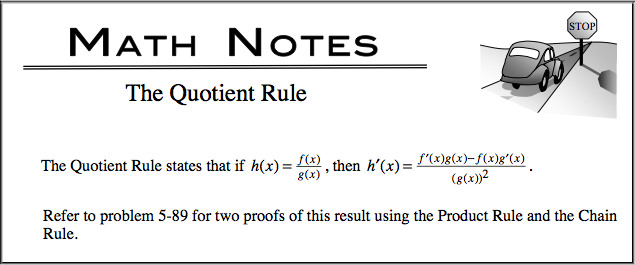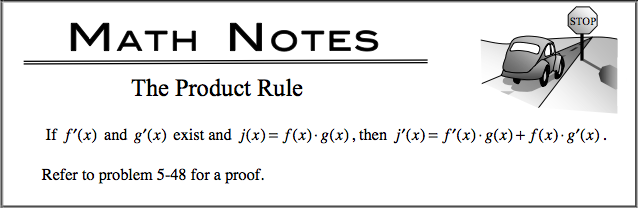### Home > CALC > Chapter 5 > Lesson 5.2.4 > Problem5-94

5-94.

Use your derivative tools to differentiate each function.

1. $\frac { d } { d x } ( \operatorname { sin } \sqrt [ 3 ] { x + 3 } )$

1. $\frac { d } { d x } ( \frac { 4 - x ^ { 2 } } { x ^ { 3 } - 2 } )$

1. $\frac { d } { d x } ( \frac { 1 } { \operatorname { cos } x } )$

1. $\frac { d } { d x } ( ( 6 x - 1 ) \operatorname { sin } ( 2 x ) )$

1. $\frac { d } { d x } ( \frac { \operatorname { sin } ( 3 x ) } { 2 x ^ { 3 } } )$

1. $\frac { d } { d x } ( \sqrt { \operatorname { sin } ^ { 2 } x + \operatorname { cos } ^ { 2 } x } )$

All of these derivatives can be found by using the Power, Product, Quotient or Chain Rule. In some cases, you will have to combine more than one rule. Be careful though! Some expressions should be simplified before differentiating, such as parts (c) and (f).